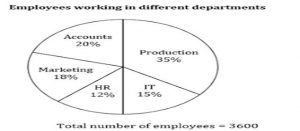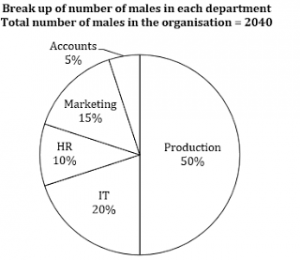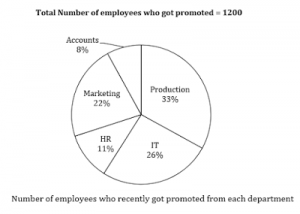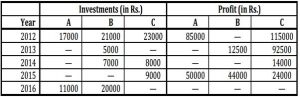## Data Interpretation for SBI PO : Set 05

Direction.1-10) Study the given pie-charts carefully to answer the questions that follow.

Break up of number of employees working in different departments of an organization, the number of males and the number of employees who recently got promoted in each department. Break up of employees working in different departments1) If half of the number of employees who got promoted from the IT department were males, what was the approximate percentage of males who got promoted from the IT department?

a) 61

b) 29

c) 54

d) 42

e) 38

e) 38

Number of male employees in IT department=2040×200/100  =408

Number of promoted male employees in IT department=1/2 (1200×26/100)  = 156

∴ Required percentage =156/408×100 ≈ 38

2) What is the total number of females working in the production and marketing departments together?

a) 468

b) 812

c) 582

d) 972

e) None of these

c) 582

Number of female employees in production department= (3600×35)/100 – (2040×50)/100

=1260-1020=240

Number of female employees is marketing department=(3600×18)/100  –  (2040×15)/100

=648-306=342

∴ Required number of females = 240+342 = 582

3) How many females work in the accounts department?

a) 618

b) 592

c) 566

d) 624

e) None of these

a) 618

Number of female employees in accounts department=(3600×200)/100  – (2040×5)/100

=720-102=618

4) The total number of employees who got promoted from all the departments together was what per cent of the total number of employees working in all the departments together? (rounded off to the nearest integer)

a) 56

b) 21

c) 45

d) 33

e) 51

d) 33

Required percentage=1200/3600×100≈33%

5) The number of employees who got promoted from the HR department was what per cent of the total number of employees working in that department? (rounded off to two digits after decimal)

a) 36.18

b) 30.56

c) 47.22

d) 28.16

e) None of these

b) 30.56

Total number of employees who got promoted=1200×11/100  = 132

Total number of employees in HR department=3600×12/100  = 432

∴ Required percentage =  132/432×100= 30.56

Directions.6-10) In the following table, the investments and profit of three persons is given for different years in a joint business.Note:
1. Apart from year 2015, they invested the amounts for same period.
2. Some values are missing. You have to calculate these value per given data.
6) If the total profit in 2014 is 49000, then find the ratio of the investment of B in 2013 to the investment of A in 2014.

a) 5 : 13

b) 10 : 27

c) 15 : 11

d) 20 : 33

e) None of these

a) 5 : 13
8000 → 4000
7000 → 14/8×7000 = 12250

Then profit made by A in 2014 = 49000- (14000+12250 )
= 22750 Rs.
∴ 4000 → 8000
22750 → 8/14×22750=13000
∴ Required Ratio = 5000 : 13000= 5 : 13[/su_spoiler]

7) In year 2015 total investment of A and B is 58000, A and B invested their amount for 6 months and 4 months respectively then find the number of months that C invested his amount ?

a) 4 months

b) 6 months

c) 8 months

d) Can’t be determined

e) None of these

c) 8 months
6 × A : 4B = 50 : 44
A : B = 25 : 33
A= 25000
B= 33000
(33000×4)/9000x=44/24

X = 8 months

8) The ratio of Total profit earned by B in year 2012 the profit earned by him in the year 2016 ?

a) 3 : 11

b) 13 : 29

c) 33 : 59

d) Can’t be determined

e) None of these

d) Can’t be determined
23000 → 11500

21000 →  115000/23000×21000

Profit of B in 2012 = 105000
Since profit of all in is not given we can’t determine the required ratio.

9) Total investment made by A,B and C in 2013 was Rs75000. Profit earned by A in 2013 is approximate what % more than the investment made by C in 2013 ?

a) 131%

b) 115%

c) 120%

d) 123%

e) 149%

d) 123%
Required % =(82500-37000)/37000×100

= 123%
10) Total profit earned by all in 2016 is 445500 Rs. and the ratio of investment made by A and B together and investment made by B and C together is 31 : 52. Then find the difference between the profit made by A and C in 2016 ?

a) 153000

b) 148500

c) 166000

d) 170000

e) None of these